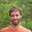# Calculation of solar radiation heat gain on opaque surfaceHi everyone

I got a quetion about equation to calculate the solar heat gain on exterior surface by energyplus. As engienering reference section 5.4.7 describes, the equation of solar heat gain can be expressred as: Qso = a(Ibcos(theta)Ss/S + IsFss +Ig*Fsg) (5.67)

The confusing part is the diffused radiation part. In section section 5.3 Sky Radiance Model, the anisotropic radiance distribution model has been introduced and sky diffuse irradiance includes sky horizon, sky dome and circumsolar region, these three parts. And if we check the equation 5.17, I can find the dome part has considered the effect of angle factor between the surface and sky.

But in equaton 5.67, the calculated sky diffuse radiance multiply sky angle factor Fss again, which really confused me. I appreciate if you got any advice for me.Many thanks

edit retag close merge delete